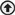# Greek Alphabet

##### Table of the Greek alphabet and what the letters are commonly used to designate.

 Name Capital Small Commonly Used to Designate Alpha A α Angles, coefficients, attenuation constant, absorption factor, area Beta B β Angles, coefficients, phase constant, luminous intensity Gamma Γ γ Complex propagation constant (cap), specific gravity, angles, electrical conductivity, propagation constant, surface tension (small) Delta Δ δ Increment or decrement (cap or small), determinant (cap), permittivity (cap), density, angles Epsilon Ε ε Dielectric constant, permittivity, base of natural logarithms, electric intensity, emissivity Zeta Ζ ζ Coordinates, coefficients Eta Η η Intrinsic impedance, efficiency, surface charge density, hysteresis, coordinates, luminous efficiency Theta Θ θ Angular phase displacement, time constant, reluctance, angles Iota Ι ι Unit vector Kappa Κ κ Susceptibility, coupling coefficient, thermal conductivity Lambda Λ λ Permeance (cap), wavelength, attenuation constant Mu Μ μ Permeability, amplification factor, prefix micro, refractive index Nu Ν ν Reluctivity, frequency, wavelength Xi Ξ ξ Coordinates Omicron Ο ο Pi Π π 3.1416 Rho Ρ ρ Resistivity, volume charge density, coordinates, reflection factor Sigma Σ σ Summation (cap), surface charge density, complex propagation constant, electrical conductivity, leakage coefficient, wavelength, deviation Tau Τ τ Time constant, volume resistivity, time-phase displacement, transmission factor, density Upsilon Υ υ Phi Φ φ Scalar potential (cap), magnetic flux, angles Chi Χ χ Electric susceptibility, angles Psi Ψ ψ Dielectric flux, phase difference, coordinates, angles Omega Ω ω Resistance in ohms (cap), solid angle (cap), angular velocity

Photonics Handbook
alphabetanglesGeneral Referencegreekback to top
©2019 Photonics Media, 100 West St., Pittsfield, MA, 01201 USA, info@photonics.comxWe deliver – right to your inbox. Subscribe FREE to our newsletters.
We use cookies to improve user experience and analyze our website traffic as stated in our Privacy Policy. By using this website, you agree to the use of cookies unless you have disabled them.